Dr. J's Maths.com
Where the techniques of Maths
are explained in simple terms.

Algebra - Indices and Powers - basic questions.
Test yourself 1.

Simplify the following expressions to evaluate your understanding of the five basic concepts underlying this topic.
Use the HINTS given if you need to.

TECHNIQUE: Be systematic in your solving of mathematics problems. Many students just begin anywhere and then wonder why they obtain incorrect answers.

In questions such as those below, move left to right by viewing the same types of terms. Often that requires you to collect the numbers first and then the first pronumeral terms together, then the second pronumerals, etc.

Multiplication and division of powers:

 1. Simplify: 3a × 2b × 5c × 4d Answer.120abcd. 2. Simplify: y3 × y5 Answer.y8. 3. Simplify: 62 × 6-5 Answer.6-3 = 1/(63) = 1/216. 4. Simplify: 2k3 × 3k4. Answer.6k7. 5. Simplify: 3x2y × 5xy3 Answer.15x3y4. 6. Simplify 7 -2 × 75 × 7 -2 Answer.7. 7. Simplify: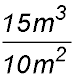. Answer.3m/2. 8.Simplify: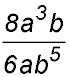. Answer.(4a2)/(3b4). 9. Simplify: 10x10 ÷ 5x6 Answer.2x4. 10. Simplify: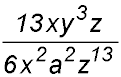. Answer.(13y3) / (x712). 11. Simplify: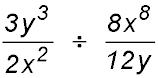Answer.(9y4) / (4x10). 12. Simplify: 3j2 × 2k3m2 × 3jkmn2 Hint.Start by multiplying the constant terms and record your answer. Then combine the j terms, then the k terms and so on. Answer.18j3 k4 m3 n2.

Powers of powers:

 13. Simplify: (k3)5 Answer.k15. 14. Simplify: (c-3)-2 Answer.c6. 15. Simplify: (x-½)-2 Answer.x. 16. Simplify fully: ((d2)-3)-1 Hint.Start by multiplying the inner exponents (2 × -3). Then multiply by the -1. So you move from inside to outside. Answer.d6. 17. Simplify fully: (3x2y3z)4 Hint.Expand the brackets by applying the outside exponent to each term in order. Answer.81x8y12z4. 18. Simplify fully: (2m3)3 × 4m5 Answer.324.

Negative powers:

 19. Rewrite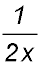with a negative index.Answer.-2/x. 20. Rewrite -2x-1 with a positive index.Answer.-2/x. 21. Simplify: (3-1)2 × 27 Answer.3. 22. Simplify. 23. Remove the brackets: (3m)-3 Answer.1/27m3. 24. Simplify: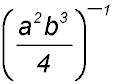. Hint.Start by removing the negative sign from outside the brackets and simply turn the inside fraction upside down.

Exponents of 1 or zero:

 25. Simplify: 50 + 1010 Answer.Sum = 2. 26. Simplify: -(4m)0 Answer.-1. 27. Simplify: - 7b0 Answer.-7. 28. Simplify: (33)0 × 52 Answer.25. 29. Simplify: 8m0 ÷ (2m)0 Hint.The first exponent of zero only applies to the m term. Answer.8. 30. Simplify: (2x3 - 4y2)1 Answer.2x3 - 4y2.

Fractional indices:

 31. Write with a fractional index: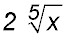. 32. Evaluate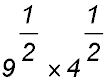Answer.6. 33. Write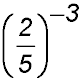with positive indices 34. Rewrite and simplify using a fractional index: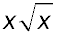35. Write in a format with radical signs:. 36. Write in a different format: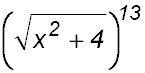.

Mixtures:

 37. Simplify: (3t)2 × 4t3 Answer.36t5. 38. Simplify:. Answer.49. 39. Simplify: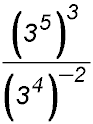Answer.323. 40. Simplify: 15m4n5 ÷ 5m2n4 Answer.3m2n. 41. Simplify: (3g3)3 ÷ (g2)4 Hint.With this question and the next, expand the brackets first by multiplying the exponents then change to negative indices for the divisions. Remember to keep working left to right in a methodological way and do not try to do too much at once. Answer.27g. 42. Simplify: (10x2y-2)3 ÷ (2x-1y3)2 Answer.250x8.